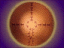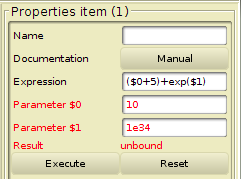ARIANE: A Visual Programming Interface

# The Item: Eval

## Definition

An Eval is an item which implements arithmetic calculus. This item has no input, no output and no mask. It has only parameters and one output result.
The number of parameters is specified during the creation of the item.

## Properties### Parameters

The parameters are named respectively:

• \$0 the first parameter;
• \$1 the second parameter;
• ...

### Arithmetic Expression

Regular arithmetic expressions use infix notations and include:

• Real values such as : 2.7, 3 et 12.7e-12 ;
• The parameter values: \$0, \$1, \$2, ...;
• Arithmetic operators: +, -, *, /, % (modulo), and ^ (power);
• Comparison operators: max(x,y), min(x,y);
• Mathematical functions: sin(x), cos(x), tan(x), asin(x), acos(x), atan(x), exp(x), ln(x), log2(x), log10(x), abs(x), sqrt(x).
Notice: Trigonometric formula are defined in radian.

(\$0*5)+exp(\$1)

### Output result

The output result is a float value.